#### Code

Caffe implementation available at https://github.com/hujie-frank/SENet

# Contribution

The authors introduce an extension called ‘Squeeze-and-Excitation’ (SE) block which should enable a network “to perform feature recalibration through which it can […] selectively emphasise […] and suppress” features.

They show how such SE-blocks improve performance on several datasets for several architectures while maintaining a reasonable network complexity (in terms of number of parameters as well as computational load).

# Proposed mechanism

The basic idea is to enforce the network to regard non-linear interdependencies between spatial features in different channels without any supervised intervention. This is achieved by reducing the output features of a transform block of the original network by a global statistic (e.g. global average pooling) and predicting a scalar weight per channel from such a vector of channel-wise (scalar) statistics.

#### SE-block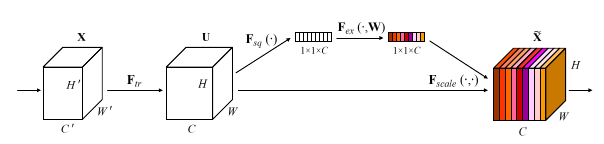• $$\mathbf{F}_{tr}$$: transform of the original network, e.g. convolutional block
• $$z_c = \mathbf{F}_{sq}(\mathbf{u}_c) = \frac{1}{H \times W} \sum_{i=1}^H \sum_{j=1}^W \, u_c(i, j)$$: squeeze operation
• $$\mathbf{s} = \mathbf{F}_{ex}(\mathbf{z}, \mathbf{W}) = \sigma(g(\mathbf{z}, \mathbf{W})) = \sigma(\mathbf{W}_2\delta(\mathbf{W}_1\mathbf{z}))$$: excitation operation ($$\delta$$: ReLU)
• $$\tilde{\mathbf{x}}_c = \mathbf{F}_{scale}(\mathbf{u}_c, s_c) = s_c \cdot \mathbf{u}_c$$: recalibration operation (i.e. rescaling)

#### Examples for extension of existing architectures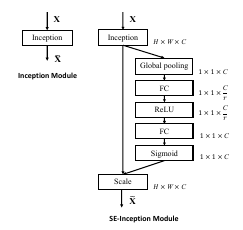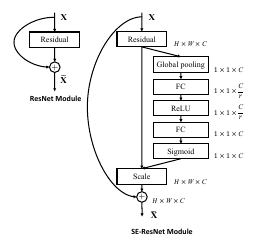# Experiments

#### Extension of existing architectures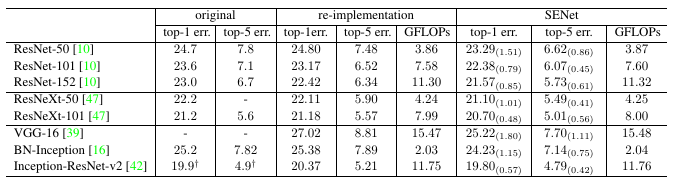• SE blocks led to improvements for all investigated base-networks on the ImageNet 2012 dataset.
• The computational overhead is small.
• The improvement is the same for different network depths.

#### Different data sets

• Similar improvements were also shown for other datasets

• Scene Classification: Places365
• Object Detection: COCO

#### Analysis of reweighting step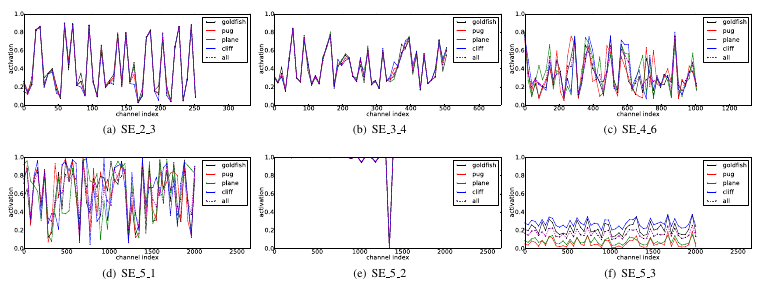Caption: Colored curves represent the average activations for different classes (computed over 50 samples for each class) plotted over channel index.

• In ‘early’ layers, the activations of the excitation step (i.e. rescaling weights) are the same among different classes.
• In ‘later’ layers (e, f), the activations are saturated.
• The reweighting among channels seems to be most significant in ‘intermediate’ layers.